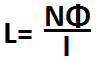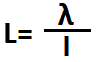﻿ Inductance Calculator

# Inductance CalculatorWb

A

nanH

The inductance calculator calculates the inductance, L, based on the product of the number of turns, N, across the conductor and the linking magnetic flux, Φ, divided by the current, I, producing the flux, according to the formula, L=(NΦ)/I

A user enters the number of turns, N, the magnetic flux, Φ, and the current, I, and the result will automatically be calculated and shown. The inductance result which is displayed above is in unit henry (H).Wb-t

A

nanH

For other conductor arrangments, inductance is given by L= λ/I.

This calculator calculates the inductance, L, based on the flux linkage, λ, divided by the current, I, producing the flux.

A user enters the flux linkage, λ, and the current, I, and the result is autoatically computed and shown. The inductance result which is displayed above is in unit henry (H).

Related Resources